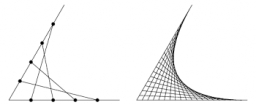# Calculate 25111

The quadratic function has the formula y = -2x²-3x + 8. Calculate the function value in points 5, -2, and ½.

y1 =  -57
y2 =  6
y3 =  6

### Step-by-step explanation:Did you find an error or inaccuracy? Feel free to write us. Thank you!

Tips for related online calculators
Are you looking for help with calculating roots of a quadratic equation?